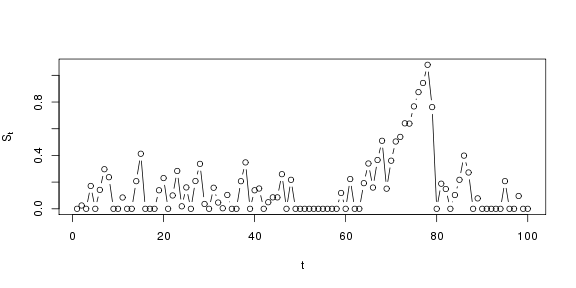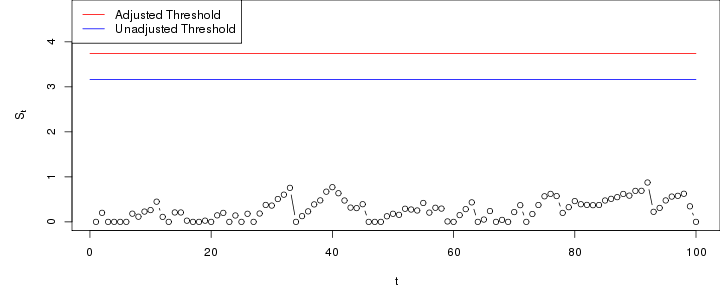# Extensions

## Extensions to other data models and estimation procedures

The framework provided in this package can be easily extended to work with other charts, other data models and other estimation procedures. The following are some examples of extensions.

## Robust estimation

Consider a CUSUM chart for observations with a normal distribution with unknown mean and standard deviation. We now illustrate how to change the estimation procedure to use the median and the mean absolute deviation (MAD) instead of the mean and the sample standard deviation. To achieve this we only need to modify one function of the existing data model, the method Pofdata that estimates the parameters .

library(spcadjust)
model <- SPCModelNormal(Delta=1)
model$Pofdata  ## function (data) ## { ## list(mu = mean(data), sd = sd(data), m = length(data)) ## } ## <environment: 0x28e30b0>  model$Pofdata <- function(data){
}


Properties of this chart can then be computed as before:

X <-  rnorm(100)
chartrobust <- new("SPCCUSUM",model=model)
SPCproperty(data=X,nrep=50,property="calARL",
chart=chartrobust,params=list(target=100),quiet=TRUE)

## 90 % CI: A threshold of 3.438 gives an in-control ARL of at least
##   100.
## Based on  50 bootstrap repetitions.


## Parametric exponential CUSUM chart

In this example we construct a CUSUM chart for exponentially distributed data with unknown rate $$\lambda$$. Such a CUSUM can be constructed by defining the updates as the log-likelihood ratio between an out of control model with rate $$\Delta\lambda$$ and an in-control model with rate $$\lambda$$. I.e.
$S_0=0, \quad S_t=\max\left(0,S_{t-1}+R_t \right)$ where $R_t=\log\left( \frac{\lambda\Delta\exp(-\lambda\Delta X_t)}{\lambda\exp(-\lambda X_t)} \right) =\log(\Delta)-\lambda(\Delta-1)X_t$ defines the updates.

We choose to use parametric bootstrapping, and the rate is estimated from past data
$$X_{-n},\dots,X_{-1}$$ by the maximum likelihood estimator $$\hat\lambda=n/\sum_{i=1}^nX_{-i}$$.

To implement this CUSUM we need to define a new data model object of class SPCDataModel. The following code does this.

SPCModelExponential=function(Delta=1.25){
structure(
list(
Pofdata=function(data){
list(lambda=1/mean(data), n=length(data))
},
xiofP=function(P) P$lambda, resample=function(P) rexp(P$n,rate=P$lambda), getcdfupdates=function(P, xi) { function(x){ if(Delta<1) pmax(0,1-exp(-P$lambda*(x-log(Delta))/(xi*(1-Delta))))
else
pmin(1,exp(-P\$lambda*(log(Delta)-x)/(xi*(Delta-1))))
}
},
class="SPCDataModel")
}


In the above code Pofdata estimates the probability model, xiofP computes the parameter needed to run the chart, resample specifies the parametric bootstrap and getcdfupdates specifies the cdf of the updates when the parameter estimate xi is used in the updates.

To create a CUSUM chart with this data model we first initialise the chart.

ExpCUSUMchart=new("SPCCUSUM",model=SPCModelExponential(Delta=1.25))


The following creates some past observations, estimates that chart parameters and runs a chart for 100 steps with new observations.

X <- rexp(1000)
plot(runchart(ExpCUSUMchart, newdata=rexp(100),xi=xiofdata(ExpCUSUMchart,X)),
ylab=expression(S[t]),xlab="t",type="b")The following computes various properties of the chart. For real applications increase the number of bootstrap replications (the argument nrep) and consider using parallel processing (the argument parallel).

SPCproperty(data=X,nrep=100,property="hitprob",
chart=ExpCUSUMchart,params=list(threshold=3,nsteps=100),
covprob=c(0.5,0.9),quiet=TRUE)

## 50 % CI: A threshold of 3 gives an in-control false alarm
##   probability of at most 0.05459 within 100 steps.
## 90 % CI: A threshold of 3 gives an in-control false alarm
##   probability of at most 0.1008 within 100 steps.
## Based on  100 bootstrap repetitions.

SPCproperty(data=X,nrep=100,property="ARL",
chart=ExpCUSUMchart,params=list(threshold=3),covprob=c(0.5,0.9),quiet=TRUE)

## 50 % CI: A threshold of 3 gives an in-control ARL of at least
##   873.1.
## 90 % CI: A threshold of 3 gives an in-control ARL of at least
##   486.9.
## Based on  100 bootstrap repetitions.

SPCproperty(data=X,nrep=100,property="calARL",chart=ExpCUSUMchart,
params=list(target=1000),covprob=c(0.5,0.9),quiet=TRUE)

## 50 % CI: A threshold of 3.214 gives an in-control ARL of at least
##   1000.
## 90 % CI: A threshold of 3.982 gives an in-control ARL of at least
##   1000.
## Based on  100 bootstrap repetitions.


To make a CUSUM plot with thresholds:

cal <- SPCproperty(data=X,nrep=1000,property="calARL",chart=ExpCUSUMchart,
params=list(target=1000),quiet=TRUE,parallel=1)
S <- runchart(ExpCUSUMchart, newdata=rexp(100),xi=xiofdata(ExpCUSUMchart,X))
par(mfrow=c(1,1),mar=c(4,5,0,0))
plot(S,ylab=expression(S[t]),xlab="t",type="b",ylim=range(S,cal@res+1,cal@raw))
lines(c(0,100),rep(cal@res,2),col="red")
lines(c(0,100),rep(cal@raw,2),col="blue")